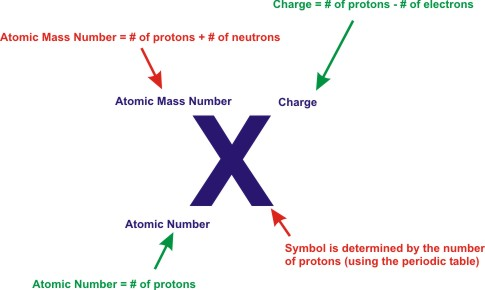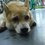# CHEMISTRY : Basic Atomic StructureSubatomic Particle Charge

   Proton                             +1

Electron                           -1

Neutron                            0


Basic Atomic Structure ~The number of protons in an atom or ion determines what element it is.

For example, if a particle has 6 protons in it, it must be carbon. (relative molar mass of carbon is 12 , so 12 - 6 neutrons= 6 protons)

The atomic number of an atom or an ion is equal to its number of protons.

Atomic Number = Number of Protons

Example: potassium (K) in the periodic table, it has an atomic number of 19, meaning that all potassium atoms and all potassium ions contain 19 protons.

Atomic Mass Number = (number of Protons) + (number of Neutrons)

Example: A particle with 6 protons and an atomic mass number of 14 has 8 neutrons.

                                                    Ions


Ions = Charged Particles

~ Ions are formed when atoms gain or lose electrons. ~Positive ions (cations) are formed when a neutral atom loses electrons. ~Negative ions (anions) are formed when a neutral atom gains electrons. ～Metallic atoms tend to lose electrons to form positive ions (also known as cations). ～Nonmetallic atoms tend to gain electrons to form negative ions (a.k.a. anions).

Charge = (number of Protons) - (number of Electrons)

Example: A particle with 34 protons and 36 electrons has a charge of -2.

                                                       Isotopes


Isotopes are atoms of the same element that have a different number of neutrons. Therefore, isotopes have the following characteristics:

Isotopes have the same atomic number (same number of protons), but a different atomic mass number (a different number of neutrons). Isotopes behave the same chemically, because they are the same element. The only difference is that one is heavier than the other, because of the additional neutrons.

For example, carbon-12 and carbon-14 are both isotopes of carbon. Carbon-12 has 6 neutrons; carbon-14 has 8 neutrons.Note by Nicole Ling
5 years, 10 months ago

This discussion board is a place to discuss our Daily Challenges and the math and science related to those challenges. Explanations are more than just a solution — they should explain the steps and thinking strategies that you used to obtain the solution. Comments should further the discussion of math and science.

When posting on Brilliant:

• Use the emojis to react to an explanation, whether you're congratulating a job well done , or just really confused .
• Ask specific questions about the challenge or the steps in somebody's explanation. Well-posed questions can add a lot to the discussion, but posting "I don't understand!" doesn't help anyone.
• Try to contribute something new to the discussion, whether it is an extension, generalization or other idea related to the challenge.

MarkdownAppears as
*italics* or _italics_ italics
**bold** or __bold__ bold
- bulleted- list
• bulleted
• list
1. numbered2. list
1. numbered
2. list
Note: you must add a full line of space before and after lists for them to show up correctly
paragraph 1paragraph 2

paragraph 1

paragraph 2

[example link](https://brilliant.org)example link
> This is a quote
This is a quote
    # I indented these lines
# 4 spaces, and now they show
# up as a code block.

print "hello world"
# I indented these lines
# 4 spaces, and now they show
# up as a code block.

print "hello world"
MathAppears as
Remember to wrap math in $$ ... $$ or $ ... $ to ensure proper formatting.
2 \times 3 $2 \times 3$
2^{34} $2^{34}$
a_{i-1} $a_{i-1}$
\frac{2}{3} $\frac{2}{3}$
\sqrt{2} $\sqrt{2}$
\sum_{i=1}^3 $\sum_{i=1}^3$
\sin \theta $\sin \theta$
\boxed{123} $\boxed{123}$

Sort by:

Basics

- 4 years, 1 month ago

Thanks a lot

- 4 years, 11 months ago

good

- 5 years ago

isobar

- 5 years, 7 months ago

# Isobars

Isobars are atoms of different elements that have the same number of nucleons (i.e. number of protons + number of neutrons). Correspondingly, isobars differ in the number of protons but have the same mass number. An example of a series of isobars would be 40-S, 40-Cl, 40-Ar, 40-K, and 40-Ca. The nuclei of these nuclides all contain 40 nucleons; however, they contain varying numbers of protons and neutrons.

# Isotones


Two elements are isotones if they have the very same neutron number, but different proton number. For example, boron-12 and carbon-13 nucleuses both contain 7 neutrons, and so are isotones.

There is also something called an isomer, however that's getting more into the structure of a chemical compound.

Just a bit of clarification on those terms. You'll know this feel when you study biology. Hahahahah...

#Let me just reiterate what those terms were.


Isotopes $\Rightarrow$ Same number of protons, but different number of neutrons.

Isobar $\Rightarrow$ Same number of (protons + neutrons) but different number of protons.

Isotone $\Rightarrow$ Same number of neutrons but different number of protons.

Also, a way to visualise this mathematically (lol) isotope, isobar and isotone are like a set of chemical elements or compounds (in the case of an isomer).

Hope this helps and no one gets confused between them. ^^ And thank you for those contributing these notes.

- 5 years, 10 months ago

Thanks for you additional notes :)))

- 5 years, 10 months ago

No worries, my pleasure. ^^

- 5 years, 10 months ago

It seems like Nicole will provide some helpful notes on Chemistry. She is my classmate and she is very interested in science especially Biology. Thumbs up for you Nicole!

- 5 years, 10 months ago

Thanks... But you are much better than me :)

- 5 years, 10 months ago